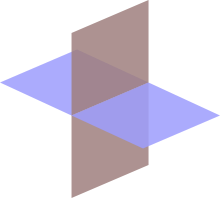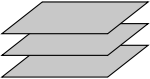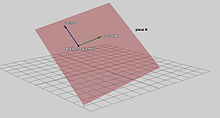# Plane (geometry)

Plane (geometry)Two intersecting planes in three-dimensional space

In mathematics, a plane is a flat, two-dimensional surface. A plane is the two dimensional analogue of a point (zero-dimensions), a line (one-dimension) and a space (three-dimensions). Planes can arise as subspaces of some higher dimensional space, as with the walls of a room, or they may enjoy an independent existence in their own right, as in the setting of Euclidean geometry.

When working in two-dimensional Euclidean space, the definite article is used, the plane, to refer to the whole space. Many fundamental tasks in mathematics, geometry, trigonometry, graph theory and graphing are performed in two-dimensional space, or in other words, in the plane.

## Euclidean geometry

Euclid set forth the first known axiomatic treatment of geometry.[citation needed] He selected a small core of undefined terms (called common notions) and postulates (or axioms) which he then used to prove various geometrical statements. Although the plane in its modern sense is not directly given a definition anywhere in the Elements, it may be thought of as part of the common notions. In his work Euclid never makes use of numbers to measure length, angle, or area. In this way the Euclidean plane is not quite the same as the Cartesian plane.Three parallel planes.

## Planes embedded in ℝ3

This section is specifically concerned with planes embedded in three dimensions: specifically, in 3.

### Properties

In three-dimensional Euclidean space, we may exploit the following facts that do not hold in higher dimensions:

• Two planes are either parallel or they intersect in a line.
• A line is either parallel to a plane, intersects it at a single point, or is contained in the plane.
• Two lines perpendicular to the same plane must be parallel to each other.
• Two planes perpendicular to the same line must be parallel to each other.

### Definition with a point and a normal vector

In a three-dimensional space, another important way of defining a plane is by specifying a point and a normal vector to the plane.

Let r0 be the position vector of some known point P0 in the plane, and let n be a nonzero vector normal to the plane. The idea is that a point P with position vector r is in the plane if and only if the vector drawn from P0 to P is perpendicular to n. Recalling that two vectors are perpendicular if and only if their dot product is zero, it follows that the desired plane can be expressed as the set of all points r such that$\bold n \cdot (\bold r-\bold r_0)=0.$

(The dot here means a dot product, not scalar multiplication.) Expanded this becomes$n_x (x-x_0)+ n_y(y-y_0)+ n_z(z-z_0)=0,\,$

which is the familiar equation for a plane.

### Define a plane with a point and two vectors lying on it

Alternatively, a plane may be described parametrically as the set of all points of the form$\bold r = \bold {r}_0 + s \bold{v} + t \bold{w},$PlaneR

where s and t range over all real numbers, v and w are given vectors defining the plane, and r0 is the vector representing the position of an arbitrary (but fixed) point on the plane. The vectors v and w can be visualized as vectors starting at r0 and pointing in different directions along the plane. Note that v and w can be perpendicular, but cannot be parallel.

### Define a plane through three points

Let p1=(x1, y1, z1), p2=(x2, y2, z2), and p3=(x3, y3, z3) be non-colinear points.

#### Method 1

The plane passing through p1, p2, and p3 can be defined as the set of all points (x,y,z) that satisfy the following determinant equations:$\begin{vmatrix} x - x_1 & y - y_1 & z - z_1 \\ x_2 - x_1 & y_2 - y_1& z_2 - z_1 \\ x_3 - x_1 & y_3 - y_1 & z_3 - z_1 \end{vmatrix} =\begin{vmatrix} x - x_1 & y - y_1 & z - z_1 \\ x - x_2 & y - y_2 & z - z_2 \\ x - x_3 & y - y_3 & z - z_3 \end{vmatrix} = 0.$

#### Method 2

To describe the plane as an equation in the form ax + by + cz + d = 0, solve the following system of equations:$\, ax_1 + by_1 + cz_1 + d = 0$$\, ax_2 + by_2 + cz_2 + d = 0$$\, ax_3 + by_3 + cz_3 + d = 0.$

This system can be solved using Cramer's Rule and basic matrix manipulations. Let$D = \begin{vmatrix} x_1 & y_1 & z_1 \\ x_2 & y_2 & z_2 \\ x_3 & y_3 & z_3 \end{vmatrix}$.

If D is non-zero (so for planes not through the origin) the values for a, b and c can be calculated as follows:$a = \frac{-d}{D} \begin{vmatrix} 1 & y_1 & z_1 \\ 1 & y_2 & z_2 \\ 1 & y_3 & z_3 \end{vmatrix}$$b = \frac{-d}{D} \begin{vmatrix} x_1 & 1 & z_1 \\ x_2 & 1 & z_2 \\ x_3 & 1 & z_3 \end{vmatrix}$$c = \frac{-d}{D} \begin{vmatrix} x_1 & y_1 & 1 \\ x_2 & y_2 & 1 \\ x_3 & y_3 & 1 \end{vmatrix}.$

These equations are parametric in d. Setting d equal to any non-zero number and substituting it into these equations will yield one solution set.

#### Method 3

This plane can also be described by the "point and a normal vector" prescription above. A suitable normal vector is given by the cross product$\bold n = ( \bold p_2 - \bold p_1 ) \times ( \bold p_3 - \bold p_1 ),$

and the point r0 can be taken to be any of the given points p1,p2 or p3.

### Distance from a point to a plane

For a plane$\Pi : ax + by + cz + d = 0\,$ and a point$\bold p_1 = (x_1,y_1,z_1)$ not necessarily lying on the plane, the shortest distance from$\bold p_1$ to the plane is$D = \frac{\left | a x_1 + b y_1 + c z_1+d \right |}{\sqrt{a^2+b^2+c^2}}.$

It follows that$\bold p_1$ lies in the plane if and only if D=0.

If$\sqrt{a^2+b^2+c^2}=1$ meaning that a, b, and c are normalized then the equation becomes$D = \ | a x_1 + b y_1 + c z_1+d | .$

### Line of intersection between two planes

The line of intersection between two planes$\Pi_1 : \bold {n}_1 \cdot \bold r = h_1$ and$\Pi_2 : \bold {n}_2 \cdot \bold r = h_2$ where$\bold {n}_i$ are normalized is given by$\bold {r} = (c_1 \bold {n}_1 + c_2 \bold {n}_2) + \lambda (\bold {n}_1 \times \bold {n}_2)$

where$c_1 = \frac{ h_1 - h_2(\bold {n}_1 \cdot \bold {n}_2) }{ 1 - (\bold {n}_1 \cdot \bold {n}_2)^2 }$$c_2 = \frac{ h_2 - h_1(\bold {n}_1 \cdot \bold {n}_2) }{ 1 - (\bold {n}_1 \cdot \bold {n}_2)^2 } .$

This is found by noticing that the line must be perpendicular to both plane normals, and so parallel to their cross product$\bold {n}_1 \times \bold {n}_2$ (this cross product is zero if and only if the planes are parallel, and are therefore non-intersecting or entirely coincident).

The remainder of the expression is arrived at by finding an arbitrary point on the line. To do so, consider that any point in space may be written as$\bold r = c_1\bold {n}_1 + c_2\bold {n}_2 + \lambda(\bold {n}_1 \times \bold {n}_2)$, since$\{ \bold {n}_1, \bold {n}_2, (\bold {n}_1 \times \bold {n}_2) \}$ is a basis. We wish to find a point which is on both planes (i.e. on their intersection), so insert this equation into each of the equations of the planes to get two simultaneous equations which can be solved for c1 and c2.

If we further assume that$\bold {n}_1$ and$\bold {n}_2$ are orthonormal then the closest point on the line of intersection to the origin is$\bold r_0 = h_1\bold {n}_1 + h_2\bold {n}_2$. If that is not the case, then a more complex procedure must be used .

### Dihedral angle

Given two intersecting planes described by$\Pi_1 : a_1 x + b_1 y + c_1 z + d_1 = 0\,$ and$\Pi_2 : a_2 x + b_2 y + c_2 z + d_2 = 0\,$, the dihedral angle between them is defined to be the angle α between their normal directions:$\cos\alpha = \hat n_1\cdot \hat n_2 = \frac{a_1 a_2 + b_1 b_2 + c_1 c_2}{\sqrt{a_1^2+b_1^2+c_1^2}\sqrt{a_2^2+b_2^2+c_2^2}}.$

## Planes in various areas of mathematics

In addition to its familiar geometric structure, with isomorphisms that are isometries with respect to the usual inner product, the plane may be viewed at various other levels of abstraction. Each level of abstraction corresponds to a specific category.

At one extreme, all geometrical and metric concepts may be dropped to leave the topological plane, which may be thought of as an idealized homotopically trivial infinite rubber sheet, which retains a notion of proximity, but has no distances. The topological plane has a concept of a linear path, but no concept of a straight line. The topological plane, or its equivalent the open disc, is the basic topological neighborhood used to construct surfaces (or 2-manifolds) classified in low-dimensional topology. Isomorphisms of the topological plane are all continuous bijections. The topological plane is the natural context for the branch of graph theory that deals with planar graphs, and results such as the four color theorem.

The plane may also be viewed as an affine space, whose isomorphisms are combinations of translations and non-singular linear maps. From this viewpoint there are no distances, but colinearity and ratios of distances on any line are preserved.

Differential geometry views a plane as a 2-dimensional real manifold, a topological plane which is provided with a differential structure. Again in this case, there is no notion of distance, but there is now a concept of smoothness of maps, for example a differentiable or smooth path (depending on the type of differential structure applied). The isomorphisms in this case are bijections with the chosen degree of differentiability.

In the opposite direction of abstraction, we may apply a compatible field structure to the geometric plane, giving rise to the complex plane and the major area of complex analysis. The complex field has only two isomorphisms that leave the real line fixed, the identity and conjugation.

In the same way as in the real case, the plane may also be viewed as the simplest, one-dimensional (over the complex numbers) complex manifold, sometimes called the complex line. However, this viewpoint contrasts sharply with the case of the plane as a 2-dimensional real manifold. The isomorphisms are all conformal bijections of the complex plane, but the only possibilities are maps that correspond to the composition of a multiplication by a complex number and a translation.

In addition, the Euclidean geometry (which has zero curvature everywhere) is not the only geometry that the plane may have. The plane may be given a spherical geometry by using the stereographic projection. This can be thought of as placing a sphere on the plane (just like a ball on the floor), removing the top point, and projecting the sphere onto the plane from this point). This is one of the projections that may be used in making a flat map of part of the Earth's surface. The resulting geometry has constant positive curvature.

Alternatively, the plane can also be given a metric which gives it constant negative curvature giving the hyperbolic plane. The latter possibility finds an application in the theory of special relativity in the simplified case where there are two spatial dimensions and one time dimension. (The hyperbolic plane is a timelike hypersurface in three-dimensional Minkowski space.)

## Topological and differential geometric notions

The one-point compactification of the plane is homeomorphic to a sphere (see stereographic projection); the open disk is homeomorphic to a sphere with the "north pole" missing; adding that point completes the (compact) sphere. The result of this compactification is a manifold referred to as the Riemann sphere or the complex projective line. The projection from the Euclidean plane to a sphere without a point is a diffeomorphism and even a conformal map.

The plane itself is homeomorphic (and diffeomorphic) to an open disk. For the Lobachevsky plane such diffeomorphism is conformal, but for the Euclidean plane it is not.

## See also

Wikimedia Foundation. 2010.

### Look at other dictionaries:

• Plane geometry — Plane Plane, a. [L. planus: cf. F. plan. See {Plan}, a.] Without elevations or depressions; even; level; flat; lying in, or constituting, a plane; as, a plane surface. [1913 Webster] Note: In science, this word (instead of plain) is almost… …   The Collaborative International Dictionary of English

• plane geometry — n. the branch of geometry dealing with plane figures …   English World dictionary

• Plane geometry — In mathematics, plane geometry may mean:*geometry of a plane, *geometry of the Euclidean plane,or sometimes a plane is any flat surface that extends without end in all directions.*geometry of a projective plane, most commonly the real projective… …   Wikipedia

• plane geometry — noun the geometry of 2 dimensional figures • Topics: ↑mathematics, ↑math, ↑maths • Hypernyms: ↑geometry * * * noun : a branch of elementary geometry that deals with plane figures * * * Math. the geometry of figures whose parts all lie in one… …   Useful english dictionary

• plane geometry — plane′ geom′etry n. math. the geometry of figures whose parts all lie in one plane • Etymology: 1740–50 …   From formal English to slang

• plane geometry — plane ge.ometry n [U] the study of lines, shapes etc that are ↑two dimensional (=with measurements in only two directions, not three) …   Dictionary of contemporary English

• plane geometry — noun Date: 1747 a branch of elementary geometry that deals with plane figures …   New Collegiate Dictionary

• plane geometry — Math. the geometry of figures whose parts all lie in one plane. [1740 50] * * * …   Universalium

• plane geometry — /pleɪn dʒiˈɒmətri/ (say playn jee omuhtree) noun the geometry of figures whose parts all lie in one plane …   Australian-English dictionary

• plane geometry — field in geometry that deals with one dimensional figures …   English contemporary dictionary

### Share the article and excerpts

##### Direct link
Do a right-click on the link above
and select “Copy Link”